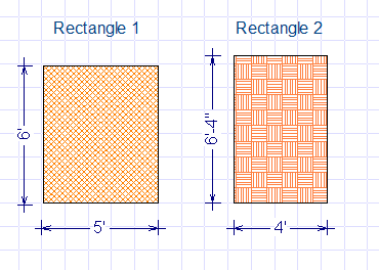Spike's Calculators

# Calculate Square Footage

### Square or Rectangular Area

To calculate the square footage of a square or rectangular area, multiply the length by the width (or height) measurement. This multiplication is done using the same units. Multiplying with mixed units will not provide the correct result. Ea. Multiplying four feet by two inches will not work.##### Example calculation;
```wall length = 15 feet 5 inches (= 15 * 12 + 5 = 185 inches)
wall height = 8 feet 2 inches (= 8 * 12 + 2 = 98 inches)```
multiply the wall length by the wall height using the same measurement units;
`185 * 98 = 18130`
`The wall has an area of 18130 square inches.`

##### Converting square inches to square feet;

To get the square feet amount, you have to know how many square inches there are in one square foot; there are 12 inches in one foot. Each side of a square foot has a length of 12 inches. To get the area of a square foot in square inches, multiply 12 by 12;

`12 * 12 = 144`
`There are 144 square inches in one square foot.`

To convert an area in square inches to square feet, divide the square inch amount by 144;
`18130 ÷ 144 = 125.9 (rounded to one decimal)`
`The wall has an area of 125.9 square feet.`

##### Estimate the number of sheets of plywood you need to cover this wall.
```wall area = 125.9 square feet rounded up to; 126 square feet. (When estimating, it is better to round up)
plywood size = 4-foot by 8-foot
```
The easiest way to do this, calculate the number of square feet in one sheet of plywood and divide the square footage of the wall by this amount;
`4 * 8 = 32`
`There are 32 square feet in one 4 x 8-foot sheet of plywood.`

`126 ÷ 32 = 3.9375 rounded to 4`
`It will take four sheets of plywood to cover this wall.`

Depending on stud layout and the fact that some of the cuttings will be useless, at times you will have to add a sheet or two, to account for waste.